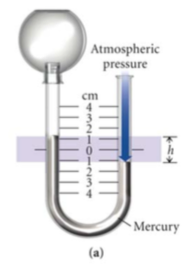# Problem: Given a barometric pressure of75 1.5 mmHg, calculate the pressure of each gas sample as indicated by the manometer.

###### FREE Expert Solution
99% (244 ratings)
###### Problem Details

Given a barometric pressure of75 1.5 mmHg, calculate the pressure of each gas sample as indicated by the manometer.What scientific concept do you need to know in order to solve this problem?

Our tutors have indicated that to solve this problem you will need to apply the Manometer concept. You can view video lessons to learn Manometer. Or if you need more Manometer practice, you can also practice Manometer practice problems.

What is the difficulty of this problem?

Our tutors rated the difficulty ofGiven a barometric pressure of75 1.5 mmHg, calculate the pre...as low difficulty.

What professor is this problem relevant for?

Based on our data, we think this problem is relevant for Professor Mack's class at CSUS.

What textbook is this problem found in?

Our data indicates that this problem or a close variation was asked in Chemistry: A Molecular Approach - Tro 3rd Edition. You can also practice Chemistry: A Molecular Approach - Tro 3rd Edition practice problems.